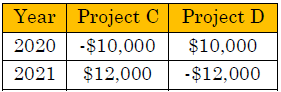top of page
Search

# How to Calculate Internal Rate of Return (IRR)

Updated: Dec 29, 2021

The Internal Rate of Return (IRR) evaluates the cost of a project. It is the rate of

return at which the present value of a project’s cash outflows and the present

value of project’s cash inflows are equal (NPV is equal to 0). If the IRR is

greater than the expected rate of return then the project should be accepted as

this project will earn more benefit to the organization.

## Use MS Excel to calculate IRR

For example, we’ll start by leveraging our original project’s cash flows…From the above statistics, we’ll construct our MS Excel spreadsheet as below…If you look at the IRR formula in cell I5, you will notice that the expected rate of

return (cell A1) is not leveraged. However, we still want to see the expected rate

of return on this sheet as a comparison point with our calculated IRR’s in cells I5

and I9.

The IRR formula just leverages the IRR function and the range of values that

represent the project’s cash flows (cells B5 through H5 and cells B9 through H9).

Since the IRR of both projects are above the 10% expected rate of return

threshold, both projects are acceptable to undertake. If you only had one choice

of project to choose from, you’d select Project A. This leads us to the below

consideration for using IRR.

## Pitfall of using IRR

A pitfall of using IRR is that it does not distinguish between the timing of

negative and positive cash flows. For example, the below cash flows represent

projects C and D.If you calculate the IRR for these projects, you will arrive at the MS Excel

Calculations as noted below...As you can see, both projects are above the expected rate of return so both

apparently can proceed. However, let’s take a look when you calculate the Net Present Value (NPV) of these projects…Now, project B does not look appealing at all and should NOT be undertaken

despite having the same IRR as project A. What is happening here?

What’s going on is that the IRR formula is not differentiating if the cash flow for

a particular year is positive (cash flow) or negative (payment) for a project.